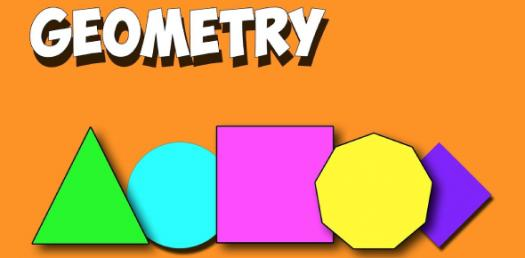# Welcome To Geometry 101 Quiz!

20 Questions | Total Attempts: 73Settings.

• 1.
Find w.
• 2.
Tell if the first triangle is acute, right, or obtuse.
• A.

Acute

• B.

Right

• C.

Obtuse

• 3.
Find x.
• 4.
Tell if the second triangle is acute, right, or obtuse.
• A.

Acute

• B.

Right

• C.

Obtuse

• 5.
Find y.
• 6.
Find w.
• 7.
Find x.
• 8.
Find y.
• 9.
Find w.
• 10.
Tell if the first triangle is acute, right, or obtuse.
• A.

Acute

• B.

Right

• C.

Obtuse

• 11.
Find x.
• 12.
Find y.
• 13.
Find x.
• 14.
Find y.
• 15.
Find z.  IGNORE THE "3" beside the 5z, it should not be there;  it is a typo.
• 16.
Find x.
• 17.
Find y.
• 18.
TRUE OR FALSE:An equilateral triangle has three sides that are the same length.
• A.

True

• B.

False

• 19.
Each angle inside an equiangular triangle measures 60 degrees.
• A.

True

• B.

False

Related Topics